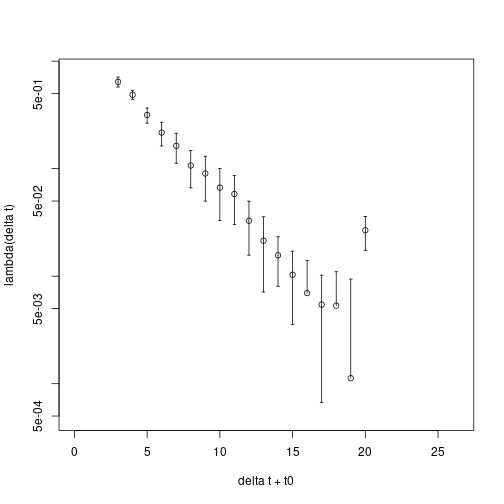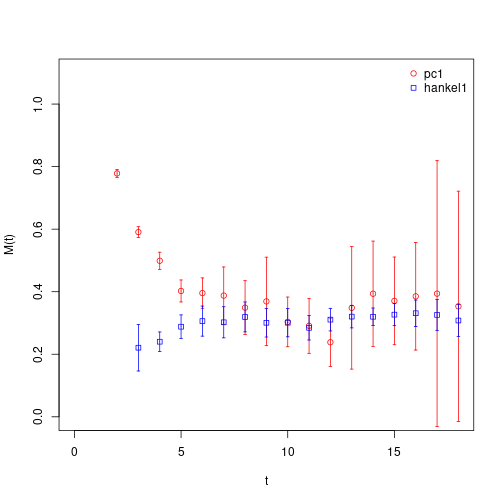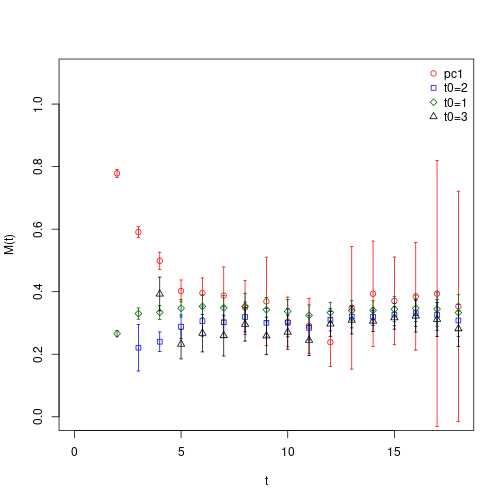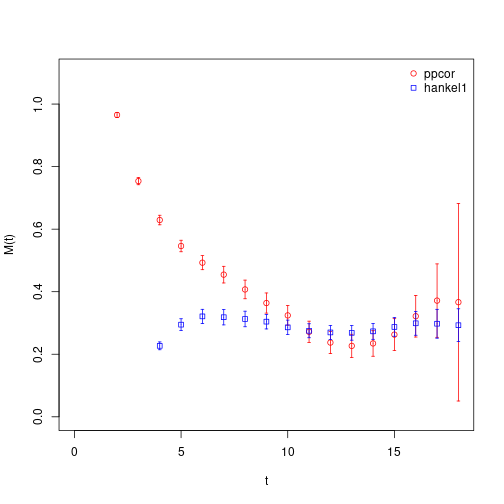# Hankel Matrix for a Correlation Function

A $$n\times n$$ Hankel matrix $$H$$ corresponding to a vector $$x=(a_0, a_1, a_2, \ldots, a_{2n-2})$$ is given by \begin{equation} H[x, n] = \begin{pmatrix} a0 & a_1 & a_2 & \ldots & a{n-1} \ a1 & a_2 & a_3 & \ldots & a{n} \ a2 & & & & \vdots \ \vdots & & & & \vdots \ a{n-1} & \ldots & & & a_{2n-2} \ \end{pmatrix} \end{equation} Lets for simplicity consider now a single correlation function $$C(t)$$ for $$t = 0, \ldots, T/2$$ with $$T$$ the temporal extent of the lattice. Define a time shift $$\delta t > 0$$, an initial time $$t_0\geq 0$$ and chose $$n < (T/2 - t_0 -\delta t)/2$$. Now define two vectors \begin{equation} \begin{split} x_1 &= (C(t_0), C(t_0+1), \ldots, C(T/2))\ x_2 &= (C(t_0+\delta t), C(t_0+\delta t+1), \ldots, C(T/2))\ \end{split} \end{equation} and the following two $$n\times n$$ Hankel matrices \begin{equation} H_1 = H[x_1, n]\,,\qquad H_2 = H[x_2, n]\,. \end{equation} With these we can define the following generalised eigenvalue problem (GEVP) \begin{equation} H_2\, v(t_0, \delta t)\ =\ H_1\, \lambda(t_0, \delta t)\, v(t_0, \delta t) \end{equation} with eigenvectors $$v(t_0, \delta t)$$ and eigenvalues $$\lambda(t_0, \delta t)$$.

If the correlator $$C(t)$$ is given by a sum of exponentials, i.e. $C(t)\ =\ \sum_{i=1}^n a_i \exp(-E_i t)\,,$ one can see that the eigenvalues $$\lambda(t_0, \delta t)$$ correspond to the exponentials as follows \begin{equation} \lambda_i(t_0, \delta t)\ =\ \exp(-E_i \delta t)\,. \end{equation} This method is known as the method of Prony \cite{prony:1795} in the literature, see also \cite{Lin:2007iq}. For an improved method see \cite{gardner:1959}.

This method is implemented in hadron as follows: we first load the sample correlator matrix, solve the $$4\times 4$$ GEVP and determine the first principal correlator:

data(correlatormatrix)
correlatormatrix <- bootstrap.cf(correlatormatrix, boot.R=99, boot.l=1, seed=132435)
correlatormatrix.gevp <- bootstrap.gevp(cf=correlatormatrix, t0=4, element.order=c(1,2,3,4))
pc1 <- gevp2cf(gevp=correlatormatrix.gevp, id=1)


Note that the data is for the pion. Next, we use the first principal correlator as input to the hankel method. For this, we call

pc1.hankel <- bootstrap.hankel(cf=pc1, t0=2, n=2)


Thus, $$n=2$$ and $$t_0=2$$ in this case. Next, we extract the lowest eigenvalue by converting into a \texttt{cf} object

hpc1 <- hankel2cf(hankel=pc1.hankel, id=1)


which looks as follows

plot(hpc1, log="y", ylab="lambda(delta t)", xlab="delta t + t0")

## Warning in xy.coords(x, y, xlabel, ylabel, log): 1 y value <= 0 omitted from
## logarithmic plotwhich could, for instance be analysed using the \texttt{matrixfit} hadron function. However, we can also cast the eigenvalues directly into effective masses, since the eigenvalues are generalisations of those.

heffectivemass1 <- hadron:::hankel2effectivemass(hankel=pc1.hankel, id=1)

## Warning in log(pc$cf.tsboot$t0): NaNs produced

## Warning in log(pc$cf.tsboot$t): NaNs produced


For comparison, we also compute the effective masses of the original principal correlator

pc1.effectivemass <- bootstrap.effectivemass(cf=pc1)


and compare in a plot

plot(pc1.effectivemass, pch=21, col="red", ylim=c(0,1.1), xlim=c(0,18),
xlab="t", ylab="M(t)")
plot(heffectivemass1, rep=TRUE, pch=22, col="blue")
legend("topright", legend=c("pc1", "hankel1"), bty="n", pch=c(21,22), col=c("red", "blue"))The result depends strongly on the choice of $$t_0$$, of course.

## Warning in log(pc$cf.tsboot$t0): NaNs produced

## Warning in log(pc$cf.tsboot$t): NaNs produced

## Warning in log(pc$cf.tsboot$t0): NaNs produced

## Warning in log(pc$cf.tsboot$t): NaNs producedOne observes increasing statistical errors, but also earlier plateaus with increasing $$t_0$$-values. We can aso apply this method to the original correlation functions directly without using the GEVP before

ppcor <- extractSingleCor.cf(cf=correlatormatrix, id=1)
ppcor.effectivemass <- bootstrap.effectivemass(cf=ppcor)
ppcor.hankel <- bootstrap.hankel(cf=ppcor, t0=3, n=2)
heffectivemass1 <- hadron:::hankel2effectivemass(hankel=ppcor.hankel, id=1)
plot(ppcor.effectivemass, pch=21, col="red", ylim=c(0,1.1), xlim=c(0,18),
xlab="t", ylab="M(t)")
plot(heffectivemass1, pch=22, col="blue", ylim=c(0,1.1), rep=TRUE)
legend("topright", legend=c("ppcor", "hankel1"), bty="n", pch=c(21,22), col=c("red", "blue"))which works not as well as the method applied to the principal correlator.

# Proof

In order to proof the GEVP relation from above, we first introduce a more general Hankel matrix $H = \begin{pmatrix} s_1 & s_2 & \ldots & s_n\ s_2 & s_3 & \ldots & \ \vdots & & & \ s_k & \ldots & & s_m\ \end{pmatrix}$ with the signal vector \begin{equation} \label{eq:signal} sk\ =\ \sum{i=1}r ci z_i{k-1}\,;\qquad z_j\ =\ e{\mathrm{i} \omega_j}\,, \end{equation} (complex frequencies $$\omega_j$$ and coefficients $$c_i$$) and $k=m-n+1 > n \geq 1\,.$ In case the sum does not start with $$z_i^0$$ for $$k=1$$ but with some $$z_i^{t_0}$$, we can simply re-define the coefficients $$c_i\to c_i' = c_i z_i^{t_0}$$ to bring $$s_k$$ into the form Eq. (\ref{eq:signal}). Define further $e\ =\ \begin{pmatrix} 1\ 1\ \vdots\ 1\ \end{pmatrix}$ and $D_c = \mathrm{diag}(c_1, \ldots, c_r)\,,\quad D_z\ =\ \mathrm{diag}(z_1, \ldots, z_r)\,.$ Then, by multiplying out, one can see that $H = \begin{pmatrix} e^T\ e^T D_z\ \vdots\ e^T D_z^{k-1}\ \end{pmatrix}\cdot D_c\cdot (e\ D_z e\ \ldots\ D_z^{n-1} e)\,.$ With this one has shown implicitly that the rank of $$H$$ is $$r$$. Now write $H\ =\ \begin{pmatrix} g_1 \ H_1\ \end{pmatrix}\ =\ \begin{pmatrix} H_2\ g_2\ \end{pmatrix}$ with [ g_1\ =\ \begin{pmatrix} s_1 & s_2 & \ldots & s_n\ \vdots & & & \vdots \ s{\delta t+1} & & & s{\delta t+n}\ \end{pmatrix} \,,\qquad g_2\ =\ \begin{pmatrix} s{k-\delta t} & & \ldots & s{m-\delta t}\ \vdots & & & \vdots \ s{k} & & & s_{m}\ \end{pmatrix}\,. ] Define two Vandermonde matrices, which have full rank $V_1\ =\ \begin{pmatrix} e^T\ e^T D_z\ \vdots\ e^T D_z^{k-1-\delta t} \end{pmatrix}\,,\qquad V_2\ =\ \begin{pmatrix} e^T\ e^T D_z\ \vdots\ e^T D_z^{n-1} \end{pmatrix}\,.$ With these we can re-write $$H$$ as $H\ =\ \begin{pmatrix} V_1 \ e^T D_z^{k-\delta t}\ \vdots\ e^T D_z^{k-1}\ \end{pmatrix} \cdot D_c\cdot V_2^T$ From this follows \begin{equation} H_2\ =\ V_1 D_c V_2T\,,\quad H_1\ =\ V_1 D_z{\delta t} D_c V_2T\,. \end{equation} Now, perform a $$QR$$ decomposition, with $$Q$$ unitary and $$R$$ upper triangular, of the Vandermonde matrices $$V_i =Q_i R_i\,,\ i=1,2$$, which is always possible due to the full rank property. This means $H_2\ =\ Q_1 R_1 D_c R_2^T Q_2^T\,,\quad H_1\ =\ Q_1 R_1 D_z^{\delta t} D_c R_2^T Q_2^T$ and, since $$D_z$$ is diagonal, by multiplying both equations with $$(R_2^T Q_2^T)^{-1}$$ from the right one obtains \begin{equation} Q_1 R_1 D_c\ =\ H_2 Q_2 R_2{-T}\ =\ D_z{-\delta t} H_1 Q_2 R_2{-T} \,. \end{equation} The last equation is the desired generalised eigenvalue relation with eigenvalues the diagonal elements of $$D_z^{\delta t}$$ and the eigenvectors the columns of the matrix $$Q_2 R_2^{-T}$$.

## Alternative

We assume $$n$$ states contributing, with $$E_k\neq 0$$ for $$k=0, \ldots, n-1$$ and all the $$E_k$$ distinct. Let $$H(t)$$ be a $$n\times n$$ Hankel matrix for $$i,j=0, 1, 2, \ldots, n-1$$ defined as \begin{equation} H{ij}(t)\ =\ \sum{k=0}{n-1} e{-E_k (t + i + j)} ck\ =\ \sum{k=0}{n-1} e{-E_k t} e{-E_k i} e{-E_k j} bk2\,, \end{equation} with $$b_k$$ the (complex) root of $$c_k$$ with positive real part. Now define \begin{equation} \chi{ki}\ =\ bk e{-E_k i}\,. \end{equation} Now introduce the dual vectors $$u_k$$ with [ (u_k, \chi_l)\ =\ \sum{i=0}{n-1} (u{k}*)_i \chi{li}\ =\ \delta{kl}\,. ] This means \begin{equation} H(t)\, u_l\ =\ \sum{k=0}{n-1} e{-E_k t}\chik \chi_k* u_l\ =\ e{-E_l t} \chi_l = e{-E_l(t-t_0)}\ e{-E_l t_0} \chi_l\ =\ e{-E_l(t-t_0)} H(t_0)\, u_l \end{equation} Thus, \begin{equation} H(t)\, u_l\ =\ e{-E_l(t-t_0)} H(t_0)\, u_l\,. \end{equation} Moreover, we get the orthogonality [ (u_l,\, H(t) u_k)\ =\ e{-E_l t}\delta{lk}\,, ] because $$H(t) u_k\propto \chi_k$$.